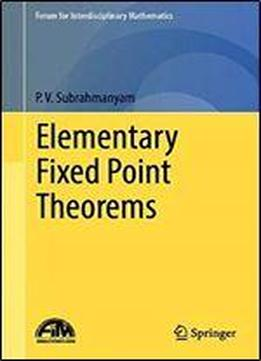# Elementary Fixed Point Theorems (forum For Interdisciplinary Mathematics) by P.V. Subrahmanyam / 2018 / English / PDF

This book provides a primary resource in basic fixed-point theorems due to Banach, Brouwer, Schauder and Tarski and their applications. Key topics covered include Sharkovsky’s theorem on periodic points, Thron’s results on the convergence of certain real iterates, Shield’s common fixed theorem for a commuting family of analytic functions and Bergweiler’s existence theorem on fixed points of the composition of certain meromorphic functions with transcendental entire functions. Generalizations of Tarski’s theorem by Merrifield and Stein and Abian’s proof of the equivalence of Bourbaki–Zermelo fixed-point theorem and the Axiom of Choice are described in the setting of posets. A detailed treatment of Ward’s theory of partially ordered topological spaces culminates in Sherrer fixed-point theorem. It elaborates Manka’s proof of the fixed-point property of arcwise connected hereditarily unicoherent continua, based on the connection he observed between set theory and fixed-point theory via a certain partial order. Contraction principle is provided with two proofs: one due to Palais and the other due to Barranga. Applications of the contraction principle include the proofs of algebraic Weierstrass preparation theorem, a Cauchy–Kowalevsky theorem for partial differential equations and the central limit theorem. It also provides a proof of the converse of the contraction principle due to Jachymski, a proof of fixed point theorem for continuous generalized contractions, a proof of Browder–Gohde–Kirk fixed point theorem, a proof of Stalling's generalization of Brouwer's theorem, examine Caristi's fixed point theorem, and highlights Kakutani's theorems on common fixed points and their applications.

views: 1137